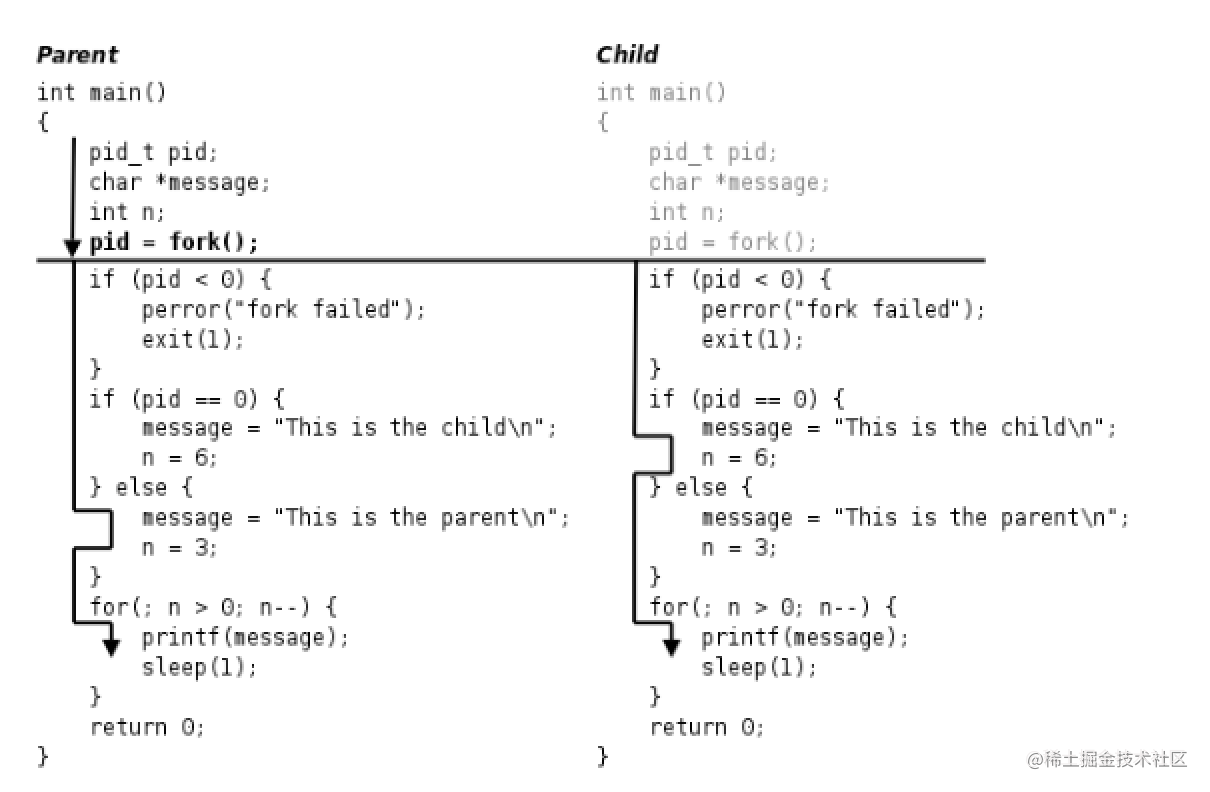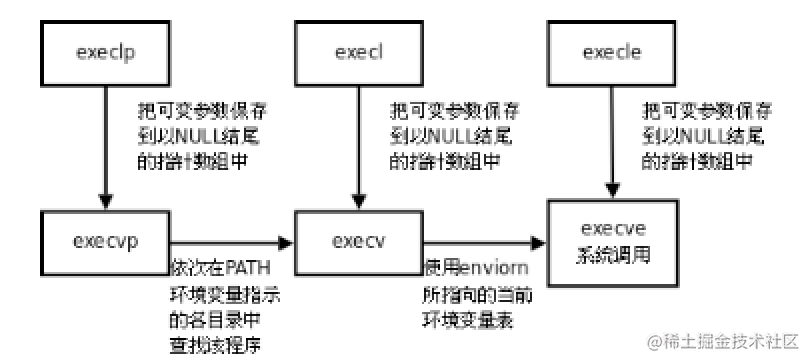# Android C++系列：Linux进程(二)「这是我参与11月更文挑战的第22天，活动详情查看：2021最后一次更文挑战」。

## 1. fork

``````#include <unistd.h>
pid_t fork(void);

• 父进程中返回子进程ID
• 子进程中返回0
• 读时共享，写时复制
``````#include <sys/types.h>
#include <unistd.h>
#include <stdio.h>
#include <stdlib.h>
int main(void) {
pid_t pid;
char *message;
int n;
pid = fork();
if (pid < 0) {
perror("fork failed");
exit(1);
}
if (pid == 0) {
message = "This is the child\n";
n = 6;
} else {
message = "This is the parent\n";
n = 3;
}
for(; n > 0; n--) {
printf(message);
sleep(1);
}
return 0;
}### 1.1 进程相关函数

``````#include <sys/types.h> #include <unistd.h>
pid_t getpid(void); //返回调用进程的PID号
pid_t getppid(void); //返回调用进程父进程的PID号

getpid/gteppid

``````#include <unistd.h>
#include <sys/types.h>
uid_t getuid(void); //返回实际用户ID
uid_t geteuid(void); //返回有效用户ID

getuid

``````#include <unistd.h>
#include <sys/types.h>
gid_t getgid(void); //返回实际用户组ID
gid_t getegid(void); //返回有效用户组ID

getgid

vfork

• 用于fork后马上调用exec函数
• 父子进程，共用同一地址空间,子进程如果没有马上exec而是修改了父进程出得到的变量值，此修改会在父进程中生效
• 设计初衷，提高系统效率，减少不必要的开销
• 现在fork已经具备读时共享写时复制机制，vfork逐渐废弃

## 2. exec族

``````#include <unistd.h>
int execl(const char *path, const char *arg, ...);
int execlp(const char *file, const char *arg, ...);
int execle(const char *path, const char *arg, ..., char *const envp[]);
int execv(const char *path, char *const argv[]);
int execvp(const char *file, char *const argv[]);
int execve(const char *path, char *const argv[], char *const envp[]);

exec调用举例如下:

``````char *const ps_argv[] ={"ps", "-o", "pid,ppid,pgrp,session,tpgid,comm", NULL};
char *const ps_envp[] ={"PATH=/bin:/usr/bin", "TERM=console", NULL};
execl("/bin/ps", "ps", "-o", "pid,ppid,pgrp,session,tpgid,comm", NULL);
execv("/bin/ps", ps_argv);
execle("/bin/ps", "ps", "-o", "pid,ppid,pgrp,session,tpgid,comm", NULL, ps_envp); execve("/bin/ps", ps_argv, ps_envp);
execlp("ps", "ps", "-o", "pid,ppid,pgrp,session,tpgid,comm", NULL);
execvp("ps", ps_argv);``````#include <unistd.h>
#include <stdlib.h>
int main(void) {
execlp("ps", "ps", "-o", "pid,ppid,pgrp,session,tpgid,comm", NULL);
perror("exec ps");
exit(1);
}

``````/* upper.c */ #include <stdio.h>
int main(void) {
int ch;
while((ch = getchar()) != EOF) {
putchar(toupper(ch));
}
return 0;
}

``````/* wrapper.c */
#include <unistd.h>
#include <stdlib.h>
#include <stdio.h>
#include <fcntl.h>
int main(int argc, char *argv[]) {
int fd;
if (argc != 2) {
fputs("usage: wrapper file\n", stderr);
exit(1);
}
fd = open(argv, O_RDONLY);
if(fd<0) {
perror("open");
exit(1);
}
dup2(fd, STDIN_FILENO);
close(fd);
execl("./upper", "upper", NULL);
perror("exec ./upper");
exit(1);
}

wrapper程序将命令行参数当作文件名打开，将标准输入重定向到这个文件，然后调用 exec执行upper程序，这时原来打开的文件描述符仍然是打开的，upper程序只负责从标准输 入读入字符转成大写，并不关心标准输入对应的是文件还是终端。运行结果如下:

• l 命令行参数列表
• p 搜素file时使用path变量
• v 使用命令行参数数组
• e 使用环境变量数组,不使用进程原有的环境变量，设置新加载程序运行的环境变量

Android

Android